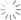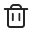English
Back
• English
• 中文繁体
• 中文简体
No matches yet
Operations too frequent. Please try again later.
Please check network settings and try again Refresh Refresh
LoadingHistory recordQuotes All >
News All >

# Asset analysis

## 1. P/L Trend

The horizontal axis represents time, and the dates at each end are the beginning and ending of the period. The vertical axis is the rate of return, and the point on the curve represents the cumulative rate of return of your total assets from the beginning of the period (0%) to the day, and compared with the index of the same period. This is not the annualized rate of return, but the cumulative rate of return from the beginning of the period to the day.

## 2. Asset Trend

The horizontal axis represents time, and the dates at each end are the beginning and the ending of the period, respectively; the vertical axis is the net asset value (NAV), and the points on the curve are the NAV of your accounts converted to the same currency each day. If it is not a trading day, the NAV equals to the NAV of the previous trading day.

## 3. Yield

Moomoo Financial Inc. uses three methods for cumulative rate of return.

### 3.1 Time-Weighted Rate of Return (TWR)

Time-weighted rate of return = [1 * (1 + the rate of return on the first day) * (1 + the rate of return on the second day)... * (1 + the rate of return on the end day)-1] * 100%

Today's Yield = Today's Income / (Yesterday's total assets +0.5*Today's net inflows)

When the account has frequent large deposits and withdrawals, the rate of return and the total P/L may be opposite, for example:

1) T day: the total assets at the beginning of the day are \$100, there is no net inflow, and the total assets at the end of T day are 150, the return on T day is positive \$50, and the return on the day is +50.00%

2) T+1 day: the total assets at the beginning of the day is 150, the net inflow of the day is 1000, and the total assets at the end of the day is 1050, the return on the day is negative \$100, and the return rate on the day = -15.38%

3) In [T,T+1], time-weighted rate of return = [(1+50.00%)×(1-15.38%)-1]*100% = +26.93%, but total P/L = +50-100 =-50

In this period, the rate of return is meaningless, please refer to the total P/L or change to other methods.

### 3.2 Money-Weighted Rate of Return (MWR/Modified Dietz)

Money-weighted yield = Total P/L / Adjusted beginning assets

Adjusted beginning assets = Beginning assets + Σ (Weight of cash flows * Cash flows)

where Weight  = (Days in period - Day of cash flow) / Days in period

This calculation improves on the accuracy of the original as it gets more precise by weighting the capital invested by the number of days it is within the portfolio. This ensures that capital which is in the fund longer has a responsibility to generate more returnl.

### 3.3 Simple Rate of Return (Original Dietz Method)

Original Dietz = Total P/L / (Beginning assets + 0.5 * Net cash flows during the period)

In an attempt to simplify the time consuming and onerous task of keep track of when external cash flows were received during the period, the formula simply multiplies the net external cash flows by 50% to assume that they were received in the middle of the period and available for investment during half of the time.

## 4. Net Cash Flows

Net cash flows = cash deposits - cash withdrawals + transfer-in stocks-transfer-out stocks

Contains two parts:

1) Cash deposit and withdrawals: refers to depositing or withdrawing funds, excluding cash seeds and gift shares;

2) Stock transfer : Calculated based on the closing price of the transfer in/out stock on the day. If a cost price is set for the transferred stock, the set price will be priority used;

## 5. Total P/L

Total P/L = Ending asset - Beginning asset - Net cash flows

Calculated based on the ending assets, beginning assets, and net inflows. It is the total profit and loss, including trading profit and loss, transaction costs, dividends,  interest costs, and exchange gains and losses.

## 6. Current Exchange Rate

The current day's exchange rate, as viewed on the Asset Trend Curve, is the historical exchange rate used to convert daily net asset values and net inflows.

## 7. Cut Off Point

The P/L data for asset analysis is calculated using 9:00 AM (UT+8:00) as the daily cut off point for P/L Calculation. For example, the profit or loss between 9:00 AM (UT+8:00) on T Day and 9:00 AM (UT+8:00) on T+1 Day is the P/L of T Day.

## 1. Is all the data on the asset analysis page updated in real time?

Assets Overview: Yes, total assets are calculated by converting NAV to the same currency at the real-time market exchange rate.

Yield Trend / Total Asset Trend / Earnings Calendar: They are updated every 1 minute (1D Trend) or every 5 minutes (5D Trend).

## 2. Why are the Total Assets and the asset value of the latest point on the real-time chart not the same?

1) Different Update Frequency: Real-time total assets are updated in almost milliseconds, but real-time charts are updated every 1 minute or every 5 minutes.

2) U.S. Stock Closing Price: After 24:00 EST, the real-time total assets use regular trading hour closing price to calculate the U.S. stock market value, but the real-time chart will continue to maintain the use of after-hours closing price calculation.

3) U.S. Stock Quotes: there are Nasdaq Basic Level 1 quotes and National Level 1 quotes, the price under these two quotes are different. The calculation price in the U.S. stock market depends on your current quote authority. Since the real-time chart is unified using the National Level 1 quotes, the total assets under the Nasdaq Basic Level 1 quotes may be deviated.

## 3. Why is today's P/L of my account different from today's P/L on Asset Analysis?

1) Different Meaning: the former is the profit and loss for trading; the latter includes not only trading, but also other fees and deductions.

2) Different Time Range: the former is calculated by using 0:00 AM of the local market as the cut off point while the latter is calculated by using 9:00 AM (UT+8:00) as the cut off point.

3) Different Exchange Rates: the former is calculated in the base currency of each account; the latter uses the real-time exchange rates to convert all currency assets into a unified currency to show the total P/L, during which there will be exchange rate differences. As a result, the total P/L on the account and P/L on the asset analysis may be slightly different.